Advertisement Remove all ads

# As the Negative Terminals of the Batteries Are Connected to A, the Net Potential Between Points a and B is − 10.3 V. - Physics

Sum

Find the potential difference V_a - V_b between the points a and b shown in each part of the figure.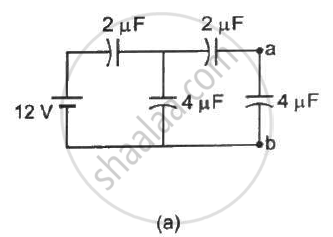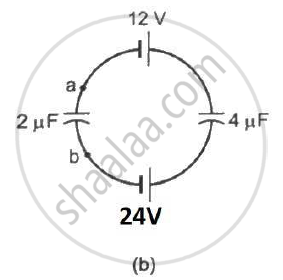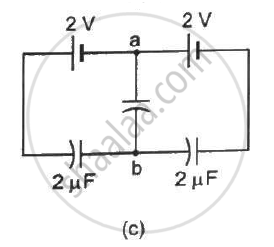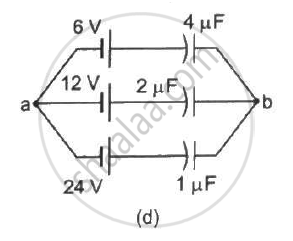Advertisement Remove all ads

#### Solution

(a) q = q_1 + q_2   ..........(1)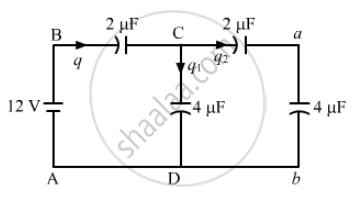On applying Kirchhoff's voltage law in the loop CabDC, we get

q_2/2 + q_2/4 - q_1/4 = 0

⇒ 2 q_2 + q_2 - q_1 = 0

⇒ 3 q_2 = q_1      .............(2)

On applying Kirchhoff's voltage law in the loop DCBAD, we get

q/2 + q_1/4 - 12 = 0

⇒ (q_1+q_2)/2 + q_1/4 - 12 = 0

⇒ 3q_1 + 2q_2 = 48        ............(3)

From eqs. (2) and (3), we get

9 q_2 + 2 q_2 = 48

⇒ 11q_2 = 48

⇒ q_2 = 48/11

Now,

V_a - V_b = q_2/(4  "uF") = 48/44 = 12/11 V

(b) Let the charge in the loop be q.

Now, on applying Kirchhoff's voltage law in the loop, we get

q/2 + q/4 - 24 +12 = 0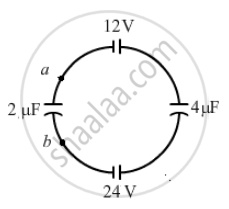⇒ (3 q)/4 = 12

⇒ q = 16  "uC"

Now ,

V_a - V_b = (-q)/(2 "uF")

⇒V_a - V_b = (-16  "uC")/(2  "uF") = -8V

(c) V_a - V_b = 2-(2-q)/(2  "uF")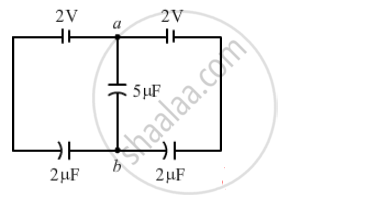In the loop,

2+2-q/2-q/2 = 0

⇒ q = 4C

therefore  V_a - V_b = 2-4/2 = 2-2 = 0 V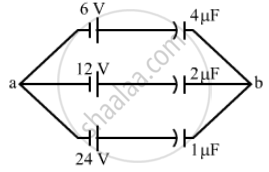Net Charge flowing through all branches, q = 24+24+24 = 72  "uC"

Net capacitance of all branches, C = 4 + 2 + 1 = 7 μF

The Total potential difference (V) between points a and b is given by

V= q/c

⇒ V = 72/7 = 10.3  "V"

As the negative terminals of the batteries are connected to a, the net potential between points a and b is -10.3 V .

Is there an error in this question or solution?
Advertisement Remove all ads

#### APPEARS IN

HC Verma Class 11, Class 12 Concepts of Physics Vol. 2
Chapter 9 Capacitors
Q 25 | Page 167
Advertisement Remove all ads

#### Video TutorialsVIEW ALL 

Advertisement Remove all ads
Share
Notifications

View all notifications

Forgot password?